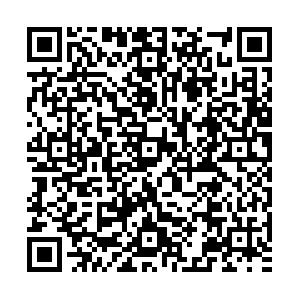# Charmonia and bottomonia in asymmetric magnetized hot nuclear matter• We investigate the mass-shift of P-wave charmonium (${\chi_c}_0$, ${\chi_c}_1$), and S and P-wave bottomonium ($\eta_b$, $\Upsilon$, ${\chi_b}_0$, and ${\chi_b}_1$) states in magnetized hot asymmetric nuclear matter using the unification of QCD sum rules (QCDSR) and the chiral $SU(3)$model. Within QCDSR, we use two approaches, i.e., the moment sum rule and the Borel sum rule. The magnetic field induced scalar gluon condensate $\left\langle \frac{\alpha_{s}}{\pi} G^a_{\mu\nu} {G^a}^{\mu\nu} \right\rangle$and the twist-2 gluon operator $\left\langle \frac{\alpha_{s}}{\pi} G^a_{\mu\sigma} {{G^a}_\nu}^{\sigma} \right\rangle$calculated in the chiral $SU(3$) model are utilised in QCD sum rules to calculate the in-medium mass-shift of the above mesons. The attractive mass-shift of these mesons is observed, which is more sensitive to magnetic field in the high density regime for charmonium, however less so for bottomonium. These results may be helpful to understand the decay of higher quarkonium states to the lower quarkonium states in asymmetric heavy ion collision experiments.
••Get Citation
Rajesh Kumar and Arvind Kumar. Charmonia and bottomonia in asymmetric magnetized hot nuclear matter[J]. Chinese Physics C, 2019, 43(12): 124109. doi: 10.1088/1674-1137/43/12/124109
Rajesh Kumar and Arvind Kumar. Charmonia and bottomonia in asymmetric magnetized hot nuclear matter[J]. Chinese Physics C, 2019, 43(12): 124109.Milestone
Revised: 2019-09-26
Article Metric

Article Views(706)
Cited by(0)
Policy on re-use
To reuse of Open Access content published by CPC, for content published under the terms of the Creative Commons Attribution 3.0 license (“CC CY”), the users don’t need to request permission to copy, distribute and display the final published version of the article and to create derivative works, subject to appropriate attribution.
###### 通讯作者: 陈斌, bchen63@163.com
• 1.

沈阳化工大学材料科学与工程学院 沈阳 110142

Title:
Email:

## Charmonia and bottomonia in asymmetric magnetized hot nuclear matter

###### Corresponding author: Arvind Kumar, iitd.arvind@gmail.com
• Department of Physics, Dr. B R Ambedkar National Institute of Technology Jalandhar, Jalandhar 144011, Punjab, India

Abstract: We investigate the mass-shift of P-wave charmonium (${\chi_c}_0$, ${\chi_c}_1$), and S and P-wave bottomonium ($\eta_b$, $\Upsilon$, ${\chi_b}_0$, and ${\chi_b}_1$) states in magnetized hot asymmetric nuclear matter using the unification of QCD sum rules (QCDSR) and the chiral $SU(3)$model. Within QCDSR, we use two approaches, i.e., the moment sum rule and the Borel sum rule. The magnetic field induced scalar gluon condensate $\left\langle \frac{\alpha_{s}}{\pi} G^a_{\mu\nu} {G^a}^{\mu\nu} \right\rangle$and the twist-2 gluon operator $\left\langle \frac{\alpha_{s}}{\pi} G^a_{\mu\sigma} {{G^a}_\nu}^{\sigma} \right\rangle$calculated in the chiral $SU(3$) model are utilised in QCD sum rules to calculate the in-medium mass-shift of the above mesons. The attractive mass-shift of these mesons is observed, which is more sensitive to magnetic field in the high density regime for charmonium, however less so for bottomonium. These results may be helpful to understand the decay of higher quarkonium states to the lower quarkonium states in asymmetric heavy ion collision experiments.

### HTML关注分享DownLoad:  Full-Size Img  PowerPoint